Related Articles
Relations and Functions
• Last Updated : 21 Feb, 2021

A function in very abstract terms can be thought of as something that will take an input and produce an output. It depends on the function what kind of input it will take and what output it will give. But, to imagine, It can be thought of as a machine or a box which gives an output for a particular value of the input.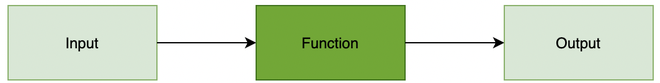Function Machine

So, where should one look for it in day to day lives?

It can be seen anywhere, for example, Weatherman takes a reading from the thermometer. The thermometer usually gives a reading in Celsius or Fahrenheit. The weatherman then converts it using some formula. That formula can be thought of as something which resides in the “Function Machine” box given in the figure above. It takes input temperature in degree Celsius and converts it into Fahrenheit. Now, can one reading of degree celsius give us two different temperature outputs in Fahrenheit? No. That’s why a rule is put on the function machine that it cannot give two outputs on taking one input.

Let’s look at it in a formal and mathematical way.

## Functions and Relation

### Cartesian product of Sets

Let’s assume A and B to be two non-empty sets, the sets of all ordered pairs (x, y) where x∈A and y∈B is called a Cartesian product of the sets.

A × B = {x, y | x∈A and y∈B}

Question: Find the cartesian products of set A ={1,2,3} and B={3,4,5}.

Answer:

Following the above definition, let Cartesian product be X,

X = A x B

= {(1,3), (1,4), (1,5), (2,3), (2,4), (2,5), (3,3), (3,4), (3,5)}

Note: Let |A| and |B| be the number of elements in set A and set B respectively. Let the number of elements in Cartesian product of the two sets be |X|. Then,

|X| = |A| x |B|

### Relation

A relation from set A to set B is a subset of the cartesian product set A x B. The subset is made up by describing a relationship between the first element and the second element of elements in A x B.

Example: R = {(1,2), (2, -3), (3,5)}

Here in the above example, set of all first elements i.e {1,2,5} is called Domain while the set of all second elements i.e {2,-3,5} is called the range of the relation.

Types of Relation

There are 8 main types of relations which include:

1. Empty Relation- There is no relation between any elements of a set.
2. Universal Relation- Every element of the set is related to each other.
3. Identity Relation- In an identity relation, every element of a set is related to itself only.
4. Inverse Relation- Inverse relation is seen when a set has elements that are inverse pairs of another set.
5. Reflexive Relation- In a reflexive relation, every element maps to itself.
6. Symmetric Relation- In asymmetric relation, if a=b is true then b=a is also true.
7. Transitive Relation- For transitive relation, if (x, y) ∈ R, (y, z) ∈ R, then (x, z) ∈ R.
8. Equivalence Relation- A relation that is symmetric, transitive, and reflexive at the same time.

### Function

A function is a special kind of relation. It is a relation in which each domain value maps only to one range value. It is denoted by ƒ:X⇒Y

What this means that it is a function from X to Y. It takes input from set X and gives the unique value from set Y as output. “X” is called the domain of the function while “Y” is called the co-domain.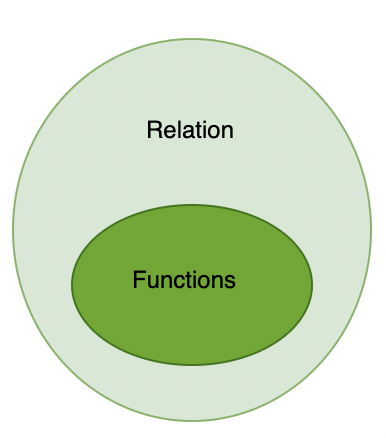All functions are relations but all relations are not functions.

As described earlier in the introduction, it can be thought of as a block/machine which runs on some formula or rule. It takes input and spits out the output.

Question: ƒ(x) = x2. Is it a function?

Answer:

Here, the function takes a number as input. So its domain is all real numbers and in the output it gives the square of that number as output. So the co-domain will be all the positive numbers.

Now, coming to the question whether this mathematical expression is function or not.

According to the definition for each value of X, it should give a unique output but vice versa is not true. That is for different values of x it can give same value as output and will still be considered as function.

For example: here, x = -2 and 2 both give the same output 4.

Since the condition is not violated, it will be considered as a function.

Some other examples of functions:

1. x3 + 1
2. sin(x), cos(x)

Types of Function:

• Identity function: The function defined by y = f (x) = x for each x ∈ R.
• Constant function: The function defined by y = f(x) = C, x ∈ R.
• Polynomial function: f(x) = anx  + an-1xn-1  + ….. + a0
• Rational function: These are function of the form p(x)/q(x).

What makes a relation a function?

A relation in which an element is mapped to only range value is called a function. To determine if a relation is a function, we just need to make sure that no element has two corresponding range values.

Question 1: A relation is given in the table below, find out whether this relation is a function or not.

Answer:

In this table, when x = 2, we have two corresponding values of Y. This violates the one input-one output property function. So this is not a function.

Question 2: A relation is given in the table below, find out whether this relation is a function or not.

Answer:

In the above relation, each input has only one corresponding range value. So, this relation is a function.

### Graphs of the Functions

All the functions can be plotted on a graph, with input values on the x-axis and their outputs on the y-axis. For example:

Let’s say f(x) = x, where x can be any real number. The graph for this function will look similar to the graph of y = x.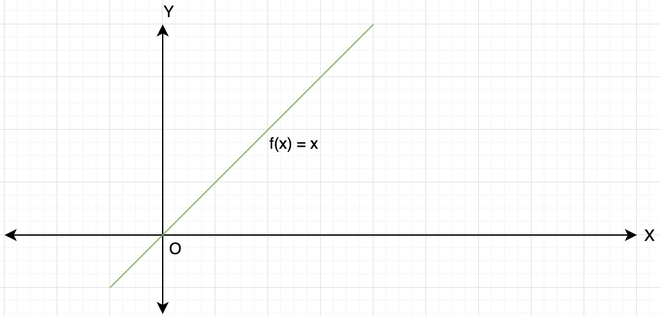f(x) = x

### How do we recognize a function from its graph?

From the condition that was described above, it’s concluded that there cannot be two values of the function at a single value of x.

So, let’s see some examples of functions and non-function.

This is a graph of f(x) = √4x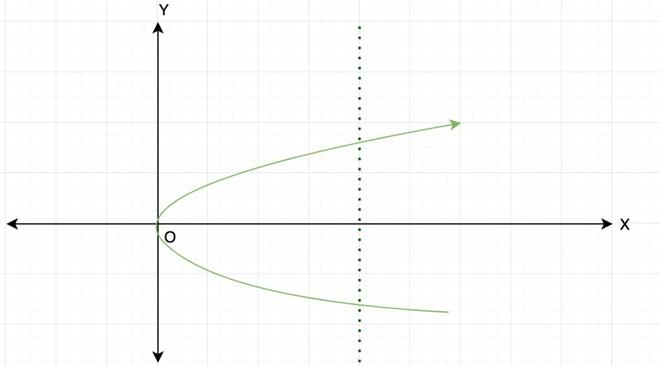In the above graph, the dashed vertical line represents a single value of x and two values of y. That means two values when given a single value of x. This violates one of the properties of the function stated above. So, this is not a function.

Let’s take another example,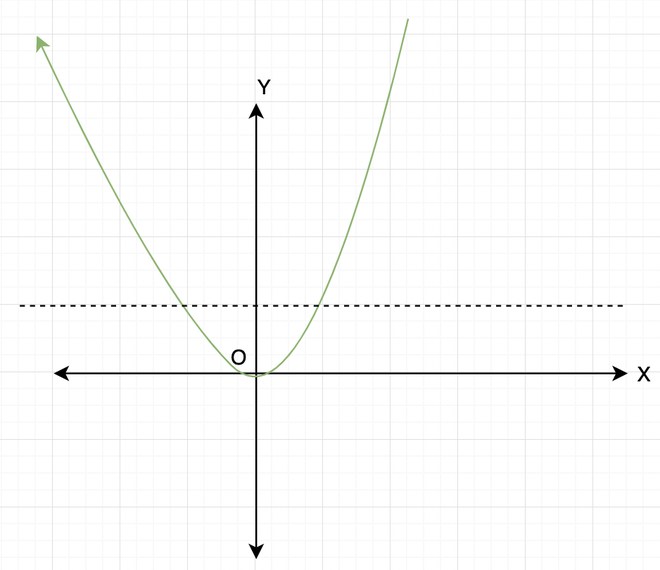In this graph above, there is no value of ‘x’ which gives two different outputs. A vertical dashed line cannot cut the graph at two places. Although a horizontal dashed line cuts the graph at two places, this indicates two inputs mapping to the same output.

### Difference between Equations and Functions

A function is an expression, a formula. An equation is an equality between two expressions.

So 8y + 1 is an expression that could be named f(y). F(y) = 8y +1 is an equation, that happens to define a function.

x2 + y2 = 4 is an equation that does not define a unique function. Its graph is a circle, which is not a function. The graph of equations represents all those points where equality is satisfied while the graph of a function represents the values outputted by function on giving different input values.

### Sample Examples

Question 1: Find out the range of function: ƒ(x) =Solution:

Since the value inside the root cannot be negative, x2 should be less than 16.

That means x ∈ [-4,4]. This is domain of the function.

For the range, let y=then y2 = 16 – x2

or x2= 16 – y2

Since x ∈ [– 4, 4]

Thus range of f = [0, 4]

Question 2: Plot the graph of function f(x) = |x|.

Solution: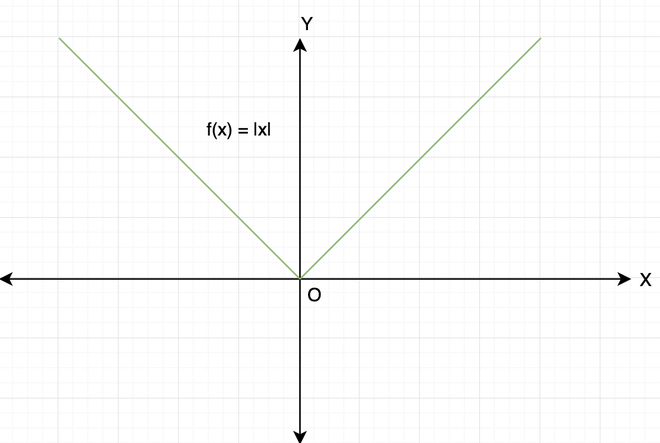It can be noticed that in this, there are multiple inputs mapping to same value of the function. For example:- x = -2 and x = 2 both give f(x) = 2.

Attention reader! Don’t stop learning now. Get hold of all the important DSA concepts with the DSA Self Paced Course at a student-friendly price and become industry ready.

My Personal Notes arrow_drop_up